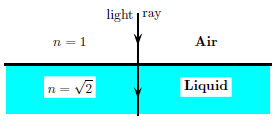# Problem: Consider a light ray which enters from air to a liquid, where the index of refraction of the liquid is given by n = √2. Denote the wavelength and the frequency in the liquid by ⋋′ and f′ and those in the air by ⋋ and f. Choose the correct pair of ratios: 1. ⋋' / ⋋ = √2, f' / f = √2 2. ⋋' / ⋋ = 1, f' / f = √2 3. ⋋' / ⋋ = 1/2, f' / f = 1 4. ⋋' / ⋋ = 1/√2, f' / f = 1 5. ⋋' / ⋋ = 2, f' / f = √2 6. ⋋' / ⋋ = 1/2, f' / f = √2 7. ⋋' / ⋋ = 1, f' / f = 1 8. ⋋' / ⋋ = 2, f' / f = 1 9. ⋋' / ⋋ = 1/√2, f' / f = √2 10. ⋋' / ⋋ = √2, f' / f = 1

###### FREE Expert Solution
94% (112 ratings)
###### Problem Details

Consider a light ray which enters from air to a liquid, where the index of refraction of the liquid is given by n = √2. Denote the wavelength and the frequency in the liquid by ⋋′ and f′ and those in the air by ⋋ and f. Choose the correct pair of ratios:

1. ⋋' / ⋋ = √2, f' / f = √2

2. ⋋' / ⋋ = 1, f' / f = √2

3. ⋋' / ⋋ = 1/2, f' / f = 1

4. ⋋' / ⋋ = 1/√2, f' / f = 1

5. ⋋' / ⋋ = 2, f' / f = √2

6. ⋋' / ⋋ = 1/2, f' / f = √2

7. ⋋' / ⋋ = 1, f' / f = 1

8. ⋋' / ⋋ = 2, f' / f = 1

9. ⋋' / ⋋ = 1/√2, f' / f = √2

10. ⋋' / ⋋ = √2, f' / f = 1